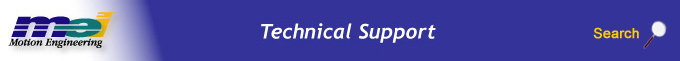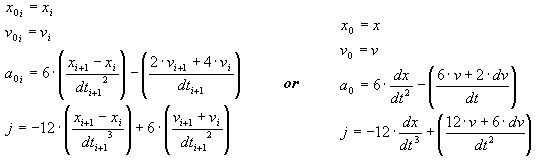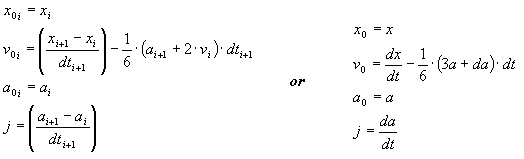.

Streaming Point Motion Type Calculations

Overview

This section is intended to provide information about how one can determine the paths (equations of motion) that will be produced by the various streaming point motion types. These equations can be used to answer several important questions such as:

 Will a certain motion cause a collision? What will the maximum acceleration be?

Since all motion is specified by constant-jerk segments of motion, it is fairly easy to calculate the maximum acceleration for any given coordinate. Since all motion segments will have accelerations that change linearly with time, simply calculate the maximum accelerations at the beginning and end of every segment. Please note that accelerations are not continuous for all motion types, so one must make two comparisons for each segment. If you are working with a multi-axis system, then this rule will also apply to a system with Cartesian axes. Although, this may not be true for non-Cartesian coordinate systems.

There is one important note about the time-delta arrays that the MEI motion types accept. Any given dt defining a point also species how long it will take to arrive at that point:PT Moves - MPIMotionTypePT

This is the most simple streaming point algorithm. This motion type will interpolate all points using constant velocity segments. The most important consequence of this motion type is that at each point defining the move, there is an instantaneous change in velocity. Therefore, one should only use PT motion when streaming points frequently—once every few servo samples. This will require several hundred, perhaps thousands of points to define a single move. If moves are to be defined using only a few points, then the other streaming point motion types should be used.

PVT Moves - MPIMotionTypePVT

PVT is the most flexible motion type because one can define both position and velocity at any given time. PVT motion guarantees continuity of velocity, but may have discontinuities in acceleration. The drawback to PVT motion is that velocities must be specified. There are a couple of major advantages of PVT motion:

 The equations of motion for any given segment are defined by the two points at the ends of the segment. PVT moves are easily appended to, without any major consequences, such as a discontinuity in velocity that are evident in some of the spline motion types.

Given xi, vi, and dti, and requiring that the velocity be continuous, it is simple to calculate the equations for motion at any given interval between the specified PVT points.Cubic Spline Moves - MPIMotionTypeSPLINE

The MPIMotionTypeSPLINE generates a "natural" cubic spline.

 A cubic spline will pass through each point defining the spline. The acceleration at the beginning and end of the motion is zero. The velocity of a cubic spline may not be zero at the beginning and end of the motion. The equations for motion of any segment depends on all points defining the spline.

Some important consequences of these characteristics are:

 When using cubic spline motion, at least two closely spaced points should be used at the beginning and at the end of a spline to help define the starting and ending velocity. Because the end-point velocities are not intuitive, there may be instantaneous changes in velocity when starting and/or appending new spline segments.

An algorithm that gives identical results to MEI's internal algorithm is given by Numerical Recipes. Numerical Recipes for C section 3.3 lists a function called spline() which outputs identical results to MEI's algorithm.